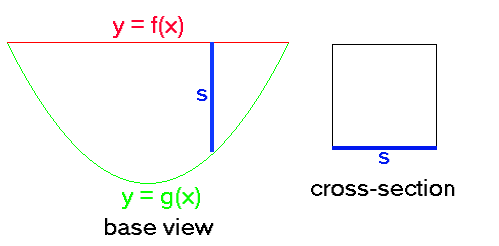The base of a certain solid is the area bounded above by the graph of $y=f(x)=9$ and below by the graph of $y=g(x)= 16 x^2$. Cross-sections perpendicular to the $x$-axis are squares. (See picture above, click for a better view.)
Use the formula to find the volume of the solid.
Note: You can get full credit for this problem by just entering the final answer (to the last question) correctly. The initial questions are meant as hints towards the final answer and also allow you the opportunity to get partial credit.
The lower limit of integration is $a$ =
The upper limit of integration is $b$ =
The side $s$ of the square cross-section is the following function of $x$:
$A(x)$=
Thus the volume of the solid is $V$ =

You can earn full credit by answering just the last part.